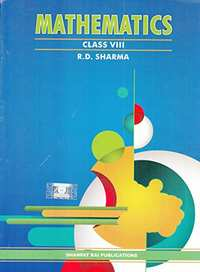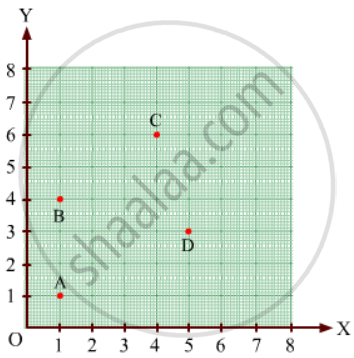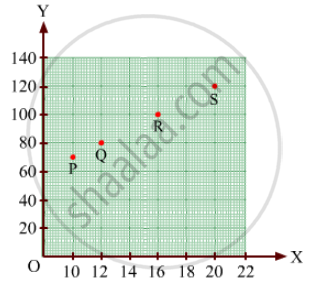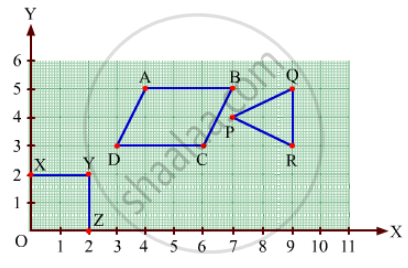Share

# RD Sharma solutions for Class 8 Mathematics chapter 27 - Introduction to Graphs

## Mathematics for Class 8 by R D Sharma (2019-2020 Session)

#### RD Sharma Mathematics Class 8 by R D Sharma (2019-2020 Session)## Chapter 27: Introduction to Graphs

Ex. 27.10Ex. 27.20

#### Chapter 27: Introduction to Graphs Exercise 27.10 solutions [Pages 5 - 7]

Ex. 27.10 | Q 1 | Page 5

Plot the points (5, 0), (5, 1), (5, 8). Do they lie on a line? What is your observation?

Ex. 27.10 | Q 2 | Page 5

Plot the points (2, 8), (7, 8) and (12, 8). Join these points in pairs. Do they lie on a line? What do you observe?

Ex. 27.10 | Q 3.1 | Page 5

Locate the points:

(1, 1), (1, 2), (1, 3), (1, 4)

Ex. 27.10 | Q 3.2 | Page 5

Locate the points:

(2, 1), (2, 2), (2, 3), (2, 4)

Ex. 27.10 | Q 3.3 | Page 5

Locate the points:

(1, 3), (2, 3), (3, 3), (4, 3)

Ex. 27.10 | Q 3.4 | Page 5

Locate the points:

(1, 4), (2, 4), (3, 4), (4, 4).

Ex. 27.10 | Q 4 | Page 6

Find the coordinates of points ABCD in Fig. 27.7.Ex. 27.10 | Q 5 | Page 6

Find the coordinates of points PQR and S in Fig. 27.8.Ex. 27.10 | Q 6 | Page 6

Write the coordinates of each of the vertices of each polygon in Fig. 27.9.Ex. 27.10 | Q 7.1 | Page 7

Decide which of the following statement is true and which is false. Give reasons for your answer.

A point whose x-coordinate is zero, will lie on the y-axis.

Ex. 27.10 | Q 7.2 | Page 7

Decide which of the following statements is true and which is false. Give reasons for your answer.

A point whose y-coordinate is zero, will lie on x-axis.

Ex. 27.10 | Q 7.3 | Page 7

Decide which of the following statements is true and which is false. Give reasons for your answer.

The coordinates of the origin are (0, 0).

Ex. 27.10 | Q 7.4 | Page 7

Decide which of the following statements is true and which is false. Give reasons for your answer.

Points whose x and y coordinates are equal, lie on a line passing through the origin.

#### Chapter 27: Introduction to Graphs Exercise 27.20 solutions [Pages 15 - 16]

Ex. 27.20 | Q 1 | Page 15

The following table shows the number of patients discharged from a hospital with HIV diagnosis in different years:

 Years: 2002 2003 2004 2005 2006 Number of patients: 150 170 195 225 230

Represent this information by a graph.

Ex. 27.20 | Q 2 | Page 15

The following table shows the amount of rice grown by a farmer in different years:

 Years: 2000 2001 2002 2003 2004 2005 2006 Rice grown (in quintals): 200 180 240 260 250 200 270

Plot a graph to illustrate this information.

Ex. 27.20 | Q 3 | Page 15

The following table gives the information regarding the number of persons employed to a piece of work and time taken to complete the work:

 Number of persons: 2 4 6 8 Time taken (in days): 12 6 4 3

Plot a graph of this information.

Ex. 27.20 | Q 4 | Page 15

The following table gives the information regarding length of a side of a square and its area:

 Length of a side (in cm): 1 2 3 4 5 Area of square (in cm2): 1 4 9 16 25

Draw a graph to illustrate this information.

Ex. 27.20 | Q 5 | Page 16

The following table shows the sales of a commodity during the years 2000 to 2006.

 Years: 2000 2001 2002 2003 2004 2005 2006 Sales (in lakhs of Rs): 1.5 1.8 2.4 3.2 5.4 7.8 8.6

Draw a graph of this information.

Ex. 27.20 | Q 6.1 | Page 16

Draw the temperature-time graph in each of the following cases:

 Time (in hours): 7:00 9:00 11:00 13:00 15:00 17:00 19:00 21:00 Temperature (°F) in: 100 101 104 102 100 99 100 98
Ex. 27.20 | Q 6.2 | Page 16

Draw the temperature-time graph in each of the following cases:

 Time (in hours): 8:00 10:00 12:00 14:00 16:00 18:00 20:00 Temperature (°F) in: 100 101 104 103 99 98 100
Ex. 27.20 | Q 7 | Page 16

Draw the velocity-time graph from the following data:

 Time (in hours): 7:00 8:00 9:00 10:00 11:00 12:00 13:00 14:00 Speed (in km/hr): 30 45 60 50 70 50 40 45
Ex. 27.20 | Q 8 | Page 16

The runs scored by a cricket team in first 15 overs are given below:

 Overs: I II III IV V VI VII VIII IX X XI XII XIII XIV XV Runs: 2 1 4 2 6 8 10 21 5 8 3 2 6 8 12

Draw the graph representing the above data in two different ways as a graph and as a bar chart.

Ex. 27.20 | Q 9 | Page 16

The runs scored by two teams A and B in first 10 overs are given below:

 Overs: I II III IV V VI VII VIII IX X Team A: 2 1 8 9 4 5 6 10 6 2 Team B: 5 6 2 10 5 6 3 4 8 10

Draw a graph depicting the data, making the graphs on the same axes in each case in two different ways as a graph and as a bar chart.

## Chapter 27: Introduction to Graphs

Ex. 27.10Ex. 27.20

#### RD Sharma Mathematics Class 8 by R D Sharma (2019-2020 Session)## RD Sharma solutions for Class 8 Mathematics chapter 27 - Introduction to Graphs

RD Sharma solutions for Class 8 Maths chapter 27 (Introduction to Graphs) include all questions with solution and detail explanation. This will clear students doubts about any question and improve application skills while preparing for board exams. The detailed, step-by-step solutions will help you understand the concepts better and clear your confusions, if any. Shaalaa.com has the CBSE Mathematics for Class 8 by R D Sharma (2019-2020 Session) solutions in a manner that help students grasp basic concepts better and faster.

Further, we at Shaalaa.com provide such solutions so that students can prepare for written exams. RD Sharma textbook solutions can be a core help for self-study and acts as a perfect self-help guidance for students.

Concepts covered in Class 8 Mathematics chapter 27 Introduction to Graphs are Concept of Bar Graph, Concept of Pie Graph (Or a Circle-graph), Concept of Histogram, Concept of a Line Graph, Some Applications, Linear Graphs - Location of a Point, Linear Graphs - Coordinates.

Using RD Sharma Class 8 solutions Introduction to Graphs exercise by students are an easy way to prepare for the exams, as they involve solutions arranged chapter-wise also page wise. The questions involved in RD Sharma Solutions are important questions that can be asked in the final exam. Maximum students of CBSE Class 8 prefer RD Sharma Textbook Solutions to score more in exam.

Get the free view of chapter 27 Introduction to Graphs Class 8 extra questions for Maths and can use Shaalaa.com to keep it handy for your exam preparation

S